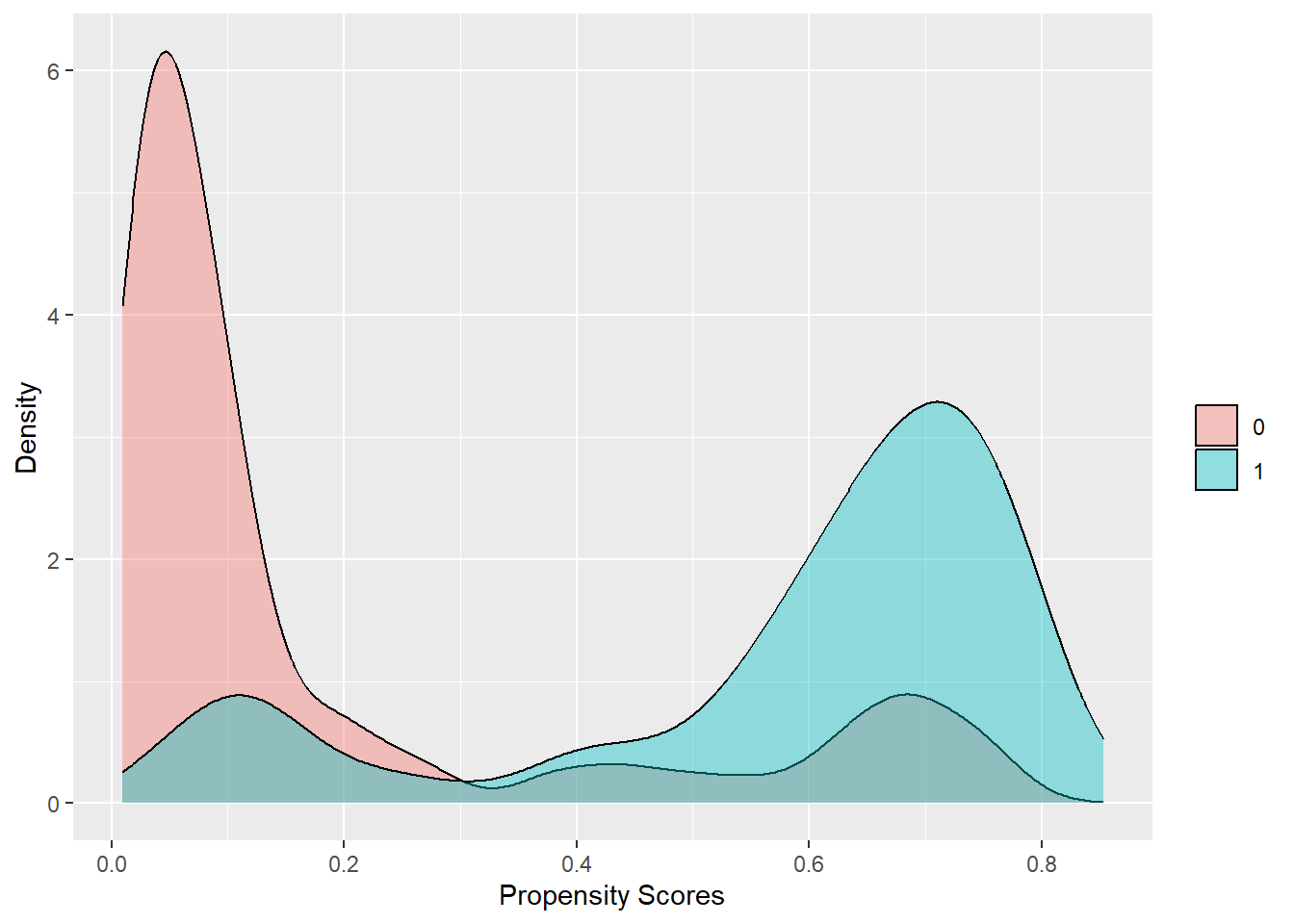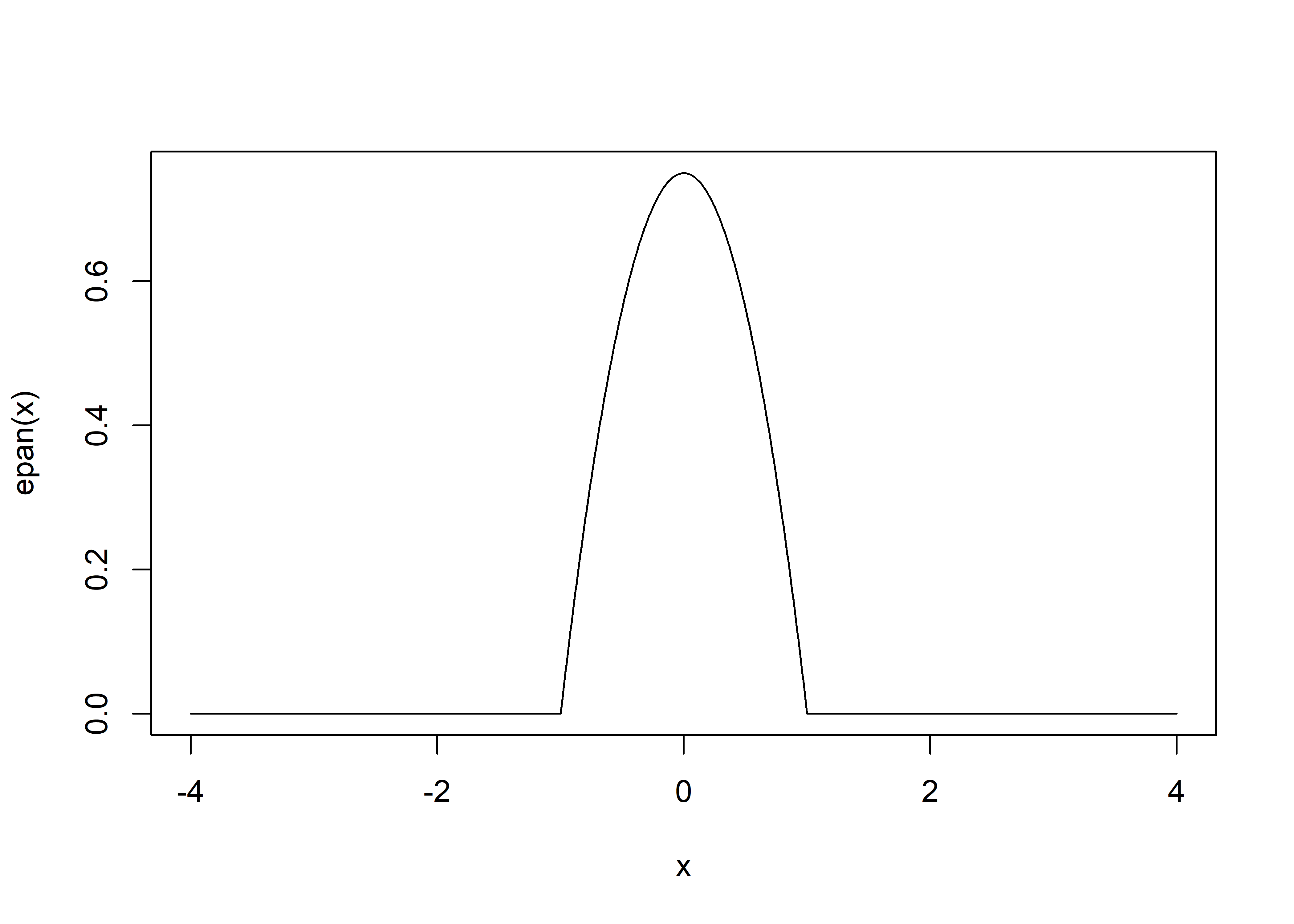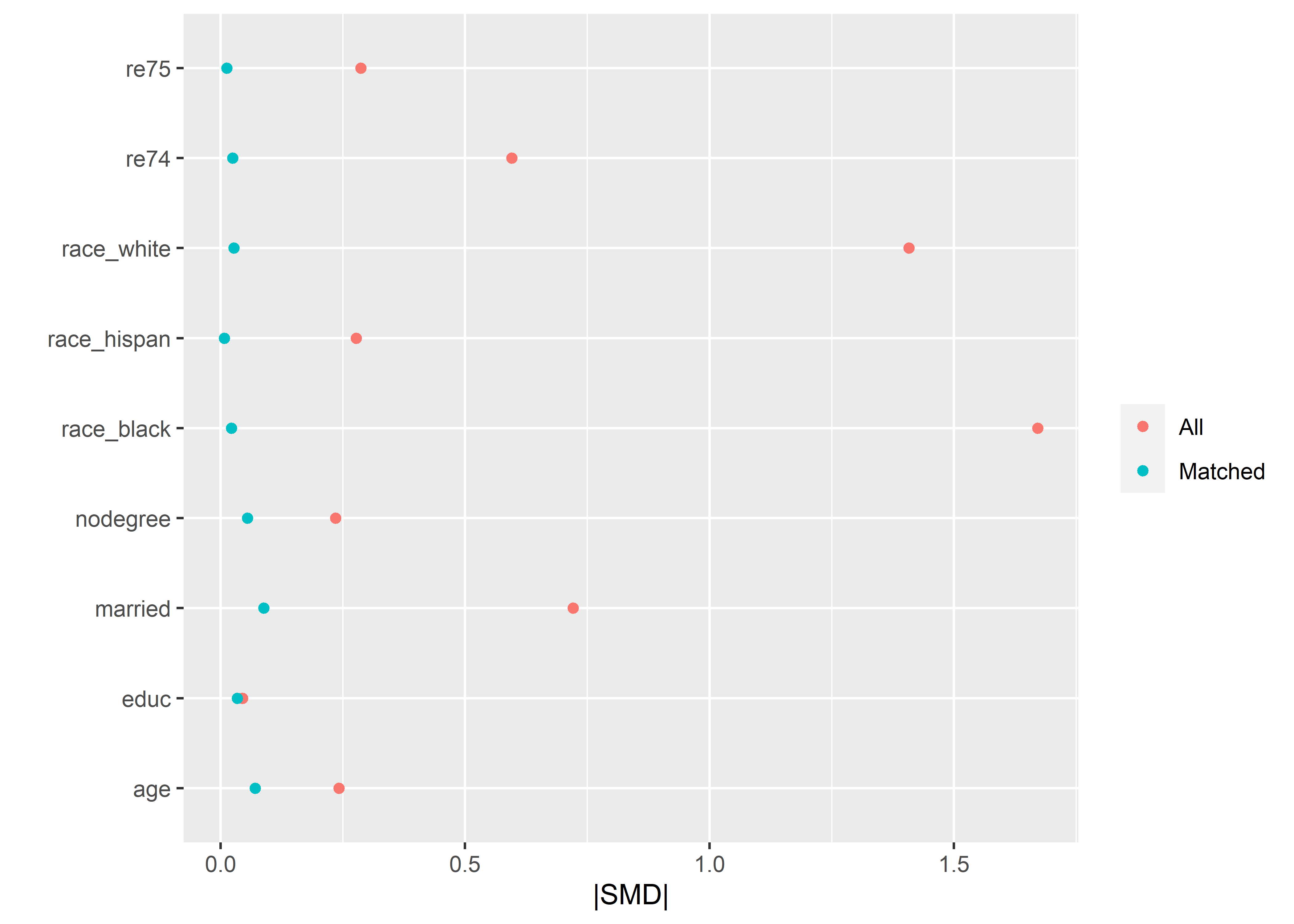library(tidyverse)
library(marginaleffects)
library(sandwich)
library(cobalt)
library(tictoc)

Use the code below at your own risk! I primarily wrote this to try to get my head around what kmatch is doing in Stata.

Sample data:

data(lalonde)

Take a peek:

head(lalonde)

## Homebaked kernel matching

First, get the propensity scores:

glm1 <- glm(
treat ~ age + educ + race + married + nodegree + re74 + re75,
data = lalonde,
family = binomial
)
lalonde$ps <- predict(glm1, type = "response") lalonde %>% ggplot(aes(x = ps, fill = factor(treat))) + geom_density(alpha = .4) + labs(x = "Propensity Scores", y = "Density", fill = "")The Epanechnikov kernel: epan <- function(x) { (3/4)*(1-x^2)*(abs(x)<=1) } curve(epan(x), -4, 4, n = 1001)I’ll want to rescale variables on an arbitrary range to [-1, 1] rescale <- function(x, in_min, in_max, out_min, out_max) { out_min + ((x - in_min)*(out_max - out_min)/(in_max - in_min)) } rescale(seq(-0.3, 0.3, .1), -0.2, 0.2, -1, 1) |> round(2) ##  -1.5 -1.0 -0.5 0.0 0.5 1.0 1.5 The tofu. This function produces a LONG dataset with control observations potentially appearing a large number of times: kmatch <- function(dat, radius, treat_var, ps_var) { res <- data.frame() # Give each observation a unique ID, for the SE calc later temp <- dat |> mutate(.id = 1:n()) treats <- temp |> filter(!!sym(treat_var) == 1) controls <- temp |> filter(!!sym(treat_var) == 0) |> arrange(!!sym(ps_var)) # hopefully speeds up filter # work through treat obs and find matches for (r in 1:nrow(treats)) { cur_row <- treats[r,] target_ps <- as.numeric(cur_row[ps_var]) # note the strictly less/greater than matches <- controls |> filter(!!sym(ps_var) > target_ps - radius & !!sym(ps_var) < target_ps + radius) |> mutate(.dist = abs(target_ps - !!sym(ps_var)), .distnorm = rescale(.dist, -radius, radius, -1, 1), .epan = epan(.distnorm), .wt = .epan/sum(.epan)) # weights sum to 1 within a class if (nrow(matches) >= 1) { cur_row$.class <- r
cur_row$.wt <- 1 # treat obs gets weight 1 matches$.class <- r

res <- bind_rows(res, cur_row, matches)
}
}

res
}

Run it:

tic()
outdat <-
kmatch(
dat = lalonde,
treat_var = "treat",
ps_var = "ps"
)
toc()
## 0.57 sec elapsed
nrow(lalonde)
##  614
nrow(outdat)
##  4696

Now aggregate the weights to make it easier to check for balance. This yields the same weights as those produced by psmatch2 and kmatch and each control observation appears only one.

outdat_agg <- outdat |>
group_by(.id) |>
summarise(.sumwt = sum(.wt))

widedat <- lalonde |>
mutate(.id = 1:n()) |>
right_join(outdat_agg)
## Joining with by = join_by(.id)

# Count

## All data

lalonde |>
group_by(treat) |>
tally()

## Matched data

outdat |>
group_by(treat) |>
summarise(unique_n = unique(.id) |> length(),
rep_n = .id |> length())

# Check balance

bal_unadj <- bal.tab(
treat ~ age + educ + race + married + nodegree + re74 + re75,
data = lalonde,
binary = "std",
continuous = "std",
s.d.denom = "pooled"
)
bal_adj <- bal.tab(
treat ~ age + educ + race + married + nodegree + re74 + re75,
data = widedat,
weights = ".sumwt",
binary = "std",
continuous = "std",
s.d.denom = "pooled"
)
summary_tab <- merge(bal_unadj$Balance |> select(-Diff.Adj,-Type), bal_adj$Balance   |> select(-Diff.Un,-Type),
by=0, all=TRUE) |>
All      = Diff.Un,
Variable = Row.names)
summary_tab
summary_tab |>
pivot_longer(cols = -Variable) |>
ggplot(aes(x = abs(value), y = factor(Variable), color = name)) +
geom_point() +
labs(y = "",
x = "|SMD|",
color = "")# Outcome model

The outcome model, as tentatively suggested by MatchIt authors for another approach using matching with replacement: “There is some evidence for an alternative approach that incorporates pair membership and adjusts for reuse of control units, though this has only been studied for survival outcomes.”

Note interaction between treat and covariates. This is a doubly-robust approach. Leave out the covariates if you don’t want that.

outfit <-
lm(
re78 ~ treat * (age + educ + race + married + nodegree + re74 + re75),
data = outdat,
weights = .wt
)

Now estimate ATT with errors clustered by subclass and ID:

avg_comparisons(
outfit,
variables = "treat",
vcov = ~ .class + .id,
newdata = subset(outdat, treat == 1),
wts = ".wt"
)

The following call replicates kmatch (no covariates, no clustering by subclass):

avg_comparisons(
lm(re78 ~ treat, data = outdat, weights = .wt),
variables = "treat",
vcov = ~ .id,
newdata = subset(outdat, treat == 1),
wts = ".wt"
)